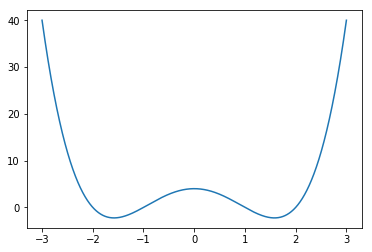### 从一元函数的角度理解梯度下降法

import matplotlib.pyplot as plt
import numpy as np
import math


f = lambda x: (x+2)*(x+1)*(x-2)*(x-1)
X = np.linspace(-3, 3, 10000)
Y = np.array([f(x) for x in X])


Y[np.argmin(Y)], X[np.argmin(Y)]

(-2.2499992119664576, -1.5808580858085808)


plt.plot(X, Y)
plt.show()$f(x)$ 为:

$$f(x)=(x+1)(x-1)(x+2)(x-2)$$

$$f’(x)=4x^3-10x$$

df = lambda x: 4*x**3-10*x
math.sqrt(5/2)

1.5811388300841898


$$x= x-\lambda f’(x)$$

lr = 0.001
x = -3
for i in range(1000):
x -= lr*df(x)
x

-1.581138831026283


$1000$ 其实就是控制 $x$ 更新的次数，对应的是深度学习中的最大迭代次数，可以看出该值基本上和 $\sqrt{\frac{5}{2}}$ 相差无几了。

### 一个好的权重初始化的意义

lr = 0.001
x = 3
for i in range(1000):
x -= lr*df(x)
x

1.581138831026283


### 动画展示梯度下降的过程

%%capture
%matplotlib inline
import numpy as np
import matplotlib.pyplot as plt

from matplotlib import animation
from IPython.display import HTML

def show_anim(X, Y, f, df, max_iterations, lr, initial_x):
fig, ax = plt.subplots()
ax.plot(X, Y)
line, = ax.plot(0, 0, 'ro')
annotation = ax.annotate("", xy=(0, 0), xytext=(0, 0), arrowprops=dict(arrowstyle="->"))
# 返回梯度下降的点，生成动画的箭头
def gradient_descent(f, df, Y, max_iterations=30, lr=0.001, x=-3):
points = [(x, f(x))]
print(lr)
for _ in range(max_iterations):
x -= lr*df(x)
points.append((x, f(x)))
arrows = []
for i in range(len(points)-1):
arrows.append((points[i], points[i+1]))
return arrows

arrows = gradient_descent(f, df, Y, max_iterations, lr, initial_x)

def init():
return line,

def update(index):
start, end = arrows[index]
line.set_data(end, end)

annotation.set_position(start)
annotation.xy = end

return line, annotation

anim = animation.FuncAnimation(fig, update, interval=500, blit=False, frames=max_iterations, init_func=init)
return anim

lr = 0.001
initial_x = -3
max_iterations = 100
anim = show_anim(X, Y, f, df, max_iterations, lr, initial_x)

HTML(anim.to_html5_video())


%%capture
%matplotlib inline
lr = 0.075
initial_x = -3
max_iterations = 100
anim = show_anim(X, Y, f, df, max_iterations, lr, initial_x)

HTML(anim.to_html5_video())


• 越在前面 loss 收敛速度越快，后面则改变幅度很小。
• 学习率过大，或导致 loss 震荡，一直达不到极小值点，虽然这里 lr=0.07 时仍然收敛了，这是因为如果 lr 过大，会直接导致超出计算机能表示的数值范围，在这里就能看出 loss 的变化幅度已经很大了。超出数值范围，对应到 caffe 中就是 Nan（not a number），这是新手容易遇到的问题。
• 学习率过小，收敛速度慢。